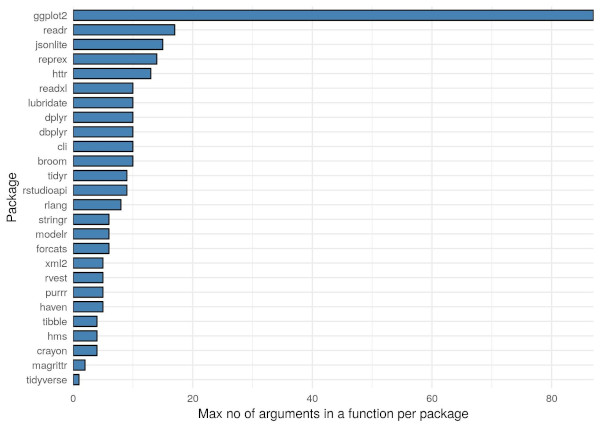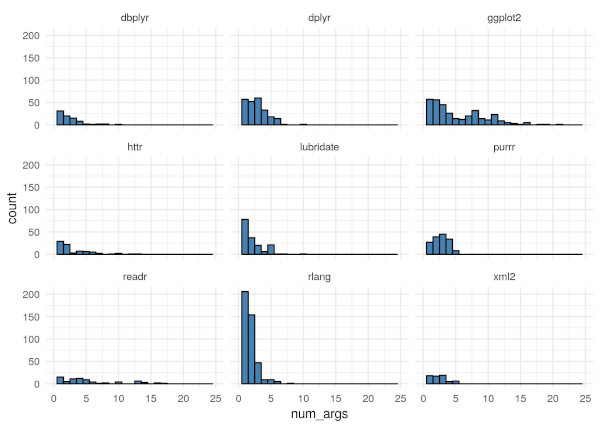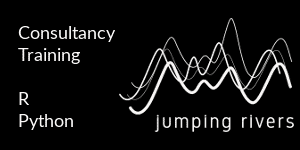GitHub

# Counting Arguments in the Tidyverse

Published: December 5, 2019Before we start anything, I’d like to mention that most of the hard work came from nsaunders and his great blog post Idle thoughts lead to R internals: how to count function arguments.

Let’s get started. The aim of this blog is to capture the number of arguments present in each function with packages of the {tidyverse}. First we need to load the necessary packages

``````library("tidyverse")
library("tidytext")
``````

Now we need to grab the relevant {tidyverse} packages

``````tpkg = tidyverse_packages()
##  "broom"  "cli"  "crayon"  "dplyr"  "dbplyr"  "forcats"
``````

We’ve had to reset the 17th element to {readxl} as it gets loaded as `readxl\n(>=`, which breaks the next block of code. Now we also need to load in the tidyverse packages. Doing this one by one would be a pain, so I’ve used `map()`

``````map(tpkg, library, character.only = TRUE)
``````

Now for the actual analysis I’m just going to whack the full code in now, then go through it line by line.

``````pkg = tpkg %>%
as_tibble() %>%
rename(package = value) %>%
rowwise() %>%
mutate(funcs = paste0(ls(paste0("package:", package)), collapse = ",")) %>%
unnest_tokens(func, funcs, token = stringr::str_split,
pattern = ",", to_lower = FALSE) %>%
filter(is.function(get(func, pos = paste0("package:", package)))) %>%
mutate(num_args = length(formalArgs(args(get(func, pos = paste0("package:", package)))))) %>%
ungroup()
``````

This is what the head of `pkg` looks like

``````head(pkg)
## # A tibble: 6 x 3
##   package func            num_args
##
## 1 broom   augment                2
## 2 broom   augment_columns        8
## 3 broom   bootstrap              3
## 4 broom   confint_tidy           4
## 5 broom   finish_glance          2
## 6 broom   fix_data_frame         3
``````

### Lines `1-4`

Lines 1-4 look like this

``````tpkg %>%
as_tibble() %>%
rename(package = value) %>%
rowwise() %>%
``````

Here we are grabbing, the tidyverse packages character vector, converting it to a tibble and renaming the column. We then use `rowwise()` so that we can work in a row-wise fashion.

### Line 5

``````mutate(funcs = paste0(ls(paste0("package:", package)), collapse = ",")) %>%
``````

To get a character vector back of the objects within a package, we do `ls("package:package_name")`. However, we want to store this as a single string, so we need to use our old friend `paste0()` to do so. We then use mutate to attach this to the data frame. Our data from now looks like this

``````## Source: local data frame [6 x 2]
## Groups:
##
## # A tibble: 6 x 2
##   package funcs
##
## 1 broom   argument_glossary,augment,augment_columns,bootstrap,column_gloss…
## 2 cli     ansi_hide_cursor,ansi_show_cursor,ansi_with_hidden_cursor,bg_bla…
## 3 crayon  %+%,bgBlack,bgBlue,bgCyan,bgGreen,bgMagenta,bgRed,bgWhite,bgYell…
## 6 forcats %>%,as_factor,fct_anon,fct_c,fct_collapse,fct_count,fct_cross,fc…
``````

### Lines 6 - 7

``````unnest_tokens(func, funcs, token = stringr::str_split,
pattern = ",", to_lower = FALSE) %>%
``````

As we’ve stored the function names as a single string, we can now apply some {tidytext} to turn our data into long data! We do this using the `unnest_tokens()` function. Here we are taking the `funcs` variable, turning it into `func` by splitting it up using `str_split()` from {stringr}. The data now looks like this

``````## Source: local data frame [6 x 2]
## Groups:
##
## # A tibble: 6 x 2
##   package func
##
## 1 broom   argument_glossary
## 2 broom   augment
## 3 broom   augment_columns
## 4 broom   bootstrap
## 5 broom   column_glossary
## 6 broom   confint_tidy
``````

### Line 8

``````filter(is.function(get(func, pos = paste0("package:", package)))) %>%
``````

Now, not every object inside a package is a function. We can use `is.function()` to test this. However, as our function names are stored as strings, we must wrap them in the `get()` function. For instance,

``````is.function("augment")
##  FALSE
is.function(get("augment"))
##  TRUE
``````

What if we have conflicts in function names? We can also specify the package our function is from, using the argument `pos`

``````is.function(get("augment", pos = "package:broom"))
##  TRUE
``````

We can then use this condition within a filter command to remove any objects that aren’t functions

### Lines 9 - end

``````mutate(num_args = length(formalArgs(args(get(func, pos = paste0("package:", package)))))) %>%
ungroup()
``````

It is possible to withdraw the arguments of a function using the `formalArgs()` function. However, this does not work on primitive functions

``````formalArgs(get("add", pos = "package:magrittr"))
## NULL
formalArgs(get("augment", pos = "package:broom"))
##  "x"   "..."
``````

We can counter act this by wrapping the function in `args()` first. This method now works for both primitives and non-primitives

``````formalArgs(args(get("add", pos = "package:magrittr")))
##  "e1" "e2"
formalArgs(args(get("augment", pos = "package:broom")))
##  "x"   "..."
``````

To work out the number of these argument we simply wrap this expression in `length()`.

### The big reveal

``````pkg %>%
arrange(desc(num_args))
## # A tibble: 2,292 x 3
##    package    func               num_args
##
##  1 ggplot2    theme                    93
##  2 ggplot2    guide_colorbar           28
##  3 ggplot2    guide_colourbar          28
##  4 ggplot2    guide_legend             21
##  5 rstudioapi launcherSubmitJob        21
##  6 ggplot2    geom_dotplot             19
##  7 ggplot2    geom_boxplot             18
## # … with 2,282 more rows
``````

So it turns out that `theme()` from {ggplot2} is king of the arguments, by a mile! The largest per package looks like thisWe’re not done there! The 9 packages with the largest sum of arguments are

``````largest = pkg %>%
group_by(package) %>%
count() %>%
arrange(desc(n)) %>%
pull(package)
largest
##  "rlang"      "ggplot2"    "dplyr"      "purrr"      "lubridate"
##  "dbplyr"     "readr"      "rstudioapi" "httr"
``````

We can plot a histogram, for each package, of the no. of arguments in each function like so..

``````pkg %>%
filter(package %in% largest) %>%
ggplot(aes(x = num_args)) +
geom_histogram(binwidth = 1, fill = "steelblue", col = "black") +
facet_wrap(~package) +
xlim(c(0, 25)) +
theme_minimal()
``````We can go a step further and retrieve the argument names as well. To do this we use the same technique as before with the functions

``````pkg %>%
rowwise() %>%
mutate(args = paste0(formalArgs(args(get(func, pos = paste0("package:", package)))),
collapse = ",")) %>%
unnest_tokens(arg, args, token = stringr::str_split,
pattern = ",", to_lower = FALSE) %>%
ungroup() %>%
count(arg) %>%
arrange(desc(n))
## # A tibble: 1,029 x 2
##    arg          n
##
##  1 ...        785
##  2 x          698
##  3 data       169
##  4 .x         120
##  5 ""         102
##  6 n           91
##  7 .f          90
##  8 position    90
##  9 mapping     79
## 10 na.rm       79
## # … with 1,019 more rows
``````

The most commonly used arguments in the tidyverse are `...` and `x` by some distance.

``````pkg %>%
rowwise() %>%
mutate(args = paste0(formalArgs(args(get(func, pos = paste0("package:", package)))),
collapse = ",")) %>%
unnest_tokens(arg, args, token = stringr::str_split,
pattern = ",", to_lower = FALSE) %>%
ungroup() %>%
group_by(package) %>%
count(arg) %>%
arrange(package, desc(n)) %>%
slice(2) %>%
arrange(desc(n))
## # A tibble: 26 x 3
## # Groups:   package 
##    package   arg         n
##
##  1 ggplot2   data      103
##  2 purrr     .x         91
##  3 dplyr     x          83
##  4 rlang     ...        64
##  6 lubridate ...        35
##  7 stringr   pattern    23
##  8 dbplyr    x          21
##  9 httr      ...        21
## 10 tidyr     ...        18
## # … with 16 more rows
``````

So you can see that `data` is the most common argument within {ggplot2}, `.x` is the most common argument within {purrr} and so on…

That’s it for this blog post. Hope you’ve enjoyed!